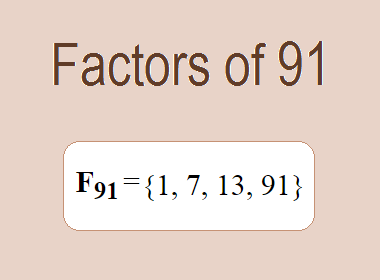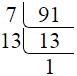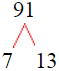# Factors of 91The factors of 91 are 1, 7, 13, and 91 i.e. F91 = {1, 7, 13, 91}. The factors of 91 are all the numbers that can divide 91 without leaving a remainder.

We can check if these numbers are factors of 91 by dividing 91 by each of them. If the result is a whole number, then the number is a factor of 91. Let's do this for each of the numbers listed above:

·        1 is a factor of 91 because 91 divided by 1 is 91.

·        7 is a factor of 91 because 91 divided by 7 is 13.

·        13 is a factor of 91 because 91 divided by 13 is 7.

·        91 is a factor of 91 because 91 divided by 91 is 1.

## How to Find Factors of 91?

1 and the number itself are the factors of every number. So, 1 and 91 are two factors of 91. To find the other factors of 91, we can start by dividing 91 by the numbers between 1 and 91. If we divide 91 by 2, we get a remainder of 1. Therefore, 2 is not a factor of 91. If we divide 91 by 3, we get a remainder of 1. Therefore, 3 is not a factor of 91.

Next, we can check if 4 is a factor of 91. If we divide 91 by 4, we get a remainder of 3. Therefore, 4 is also not a factor of 91. We can continue this process for all the possible factors of 91.

Through this process, we can find that the factors of 91 are 1, 7, 13, and 91. These are the only numbers that can divide 91 without leaving a remainder.

********************

********************

## Properties of the Factors of 91

The factors of 91 have some interesting properties. One of the properties is that the sum of the factors of 91 is equal to 112. We can see this by adding all the factors of 91 together:

1 + 7 + 13 + 91 = 112

Another property of the factors of 91 is that they are all odd numbers. This is because 91 is an odd number, and any even number cannot divide an odd number without leaving a remainder.

Another property of the factors of 91 is that the prime factors of 91 are 7 and 13.

## Applications of the Factors of 91

The factors of 91 have several applications in mathematics. One of the applications is in finding the highest common factor (HCF) of two or more numbers. The HCF is the largest factor that two or more numbers have in common. For example, to find the HCF of 91 and 65, we need to find the factors of both numbers and identify the largest factor they have in common. The factors of 91 are 1, 7, 13, and 91. The factors of 65 are 1, 5, 13, and 65. The largest factor that they have in common is 13. Therefore, the HCF of 91 and 65 is 13.

Another application of the factors of 91 is in prime factorization. Prime factorization is the process of expressing a number as the product of its prime factors. The prime factors of 91 are 7 and 13. We can express 91 as:

91 = 7 × 13

We can do prime factorization by division and factor tree method also. Here is the prime factorization of 91 by division method,91 = 7 × 13

Here is the prime factorization of 91 by the factor tree method,91 = 7 × 13

## Conclusion

The factors of 91 are the numbers that can divide 91 without leaving a remainder. The factors of 91 are 1, 7, 13, and 91. The factors of 91 have some interesting properties, such as being odd numbers and having a sum of 112. The factors of 91 have several applications in mathematics, such as finding the highest common factor and prime factorization.